# Construction Of Quadrilaterals With Given Diagonals

Before talking about the construction of quadrilaterals, let us recall what a quadrilateral is. A quadrilateral is a polygon which has 4 vertices and 4 sides enclosing 4 angles. The sum of its interior angles is 360 degrees. A quadrilateral, in general, has sides of different lengths and angles of different measures. However, squares, rectangles, etc. are special types of quadrilaterals with some of their sides and angles being equal. In this article, we will discuss the construction of quadrilaterals, where the measurements of its three sides and two diagonals are given.

## Steps to Construct Quadrilaterals (With Example)

Let us say you are required to construct a quadrilateral PQRS where the measurements are:

• QR = 7 cm
• RS = 5 cm
• PS = 4 cm
• Diagonal SQ = 9 cm
• Diagonal PR = 6 cm

For the construction of quadrilaterals with some of the measurements given, we first draw a rough figure of the quadrilateral with the given dimensions, as shown below.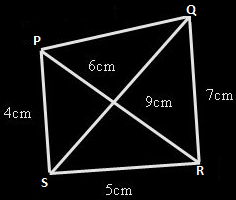Now starting with the construction, the steps are:

• Draw a line segment of length 5 cm and mark the ends as S and R.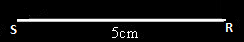• Set your compass to the radius of 6 cm and make an arc from the point R above the line segment.
• Set the compass to the radius of 4 cm and make an arc from the point S on the previous arc.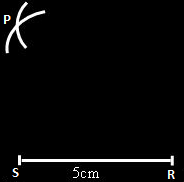• Mark the point as P where the two arc cross each other. Join the points S and P as well as R and P.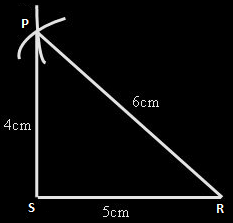• Set the compass to the radius of 9 cm and make an arc from the point S.
• Set the compass to the radius of 7 cm and make an arc from the point R on the previous arc.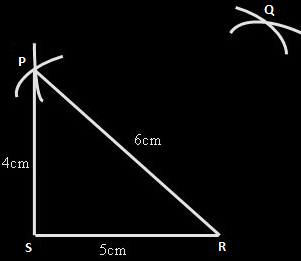• Mark the point as Q where the two arc cross each other.
• Join the points P and Q as well as Q and R.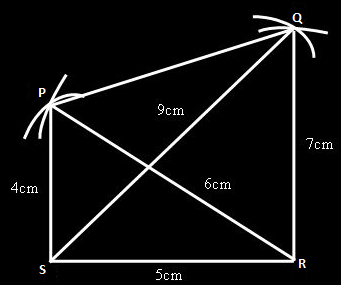You obtain the quadrilateral PQRS of the required measurements.